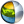Cg 3.1 Toolkit Documentation Cg / Standard Library / sqrt

## Name

sqrt - returns square root of scalars and vectors.

## Synopsis

```float  sqrt(float a);
float1 sqrt(float1 a);
float2 sqrt(float2 a);
float3 sqrt(float3 a);
float4 sqrt(float4 a);

half   sqrt(half a);
half1  sqrt(half1 a);
half2  sqrt(half2 a);
half3  sqrt(half3 a);
half4  sqrt(half4 a);

fixed  sqrt(fixed a);
fixed1 sqrt(fixed1 a);
fixed2 sqrt(fixed2 a);
fixed3 sqrt(fixed3 a);
fixed4 sqrt(fixed4 a);
```

## Parameters

a
Vector or scalar of which to determine the square root.

## Description

Returns the square root of a.

For vectors, the returned vector contains the square root of each element of the input vector.

The square root of zero is zero.

Ideally, the square root of negative values returns NaN (Not a Number).

sqrt often implemented as the reciprocal of a reciporcal squrare root approximation in older profiles.

## Reference Implementation

sqrt is best implemented as a native square root instruction, however sqrt may be implemented via a rsqrt function:

```float3 sqrt(float3 a)
{
return 1.0 / rsqrt(a);
}
```

## Profile Support

sqrt is fully supported in all profiles unless otherwise specified. sqrt is unsupported in the fp20 profile.| Cg Toolkit | Download | Release Archive | Profiles | Reference | Books | Discussions |